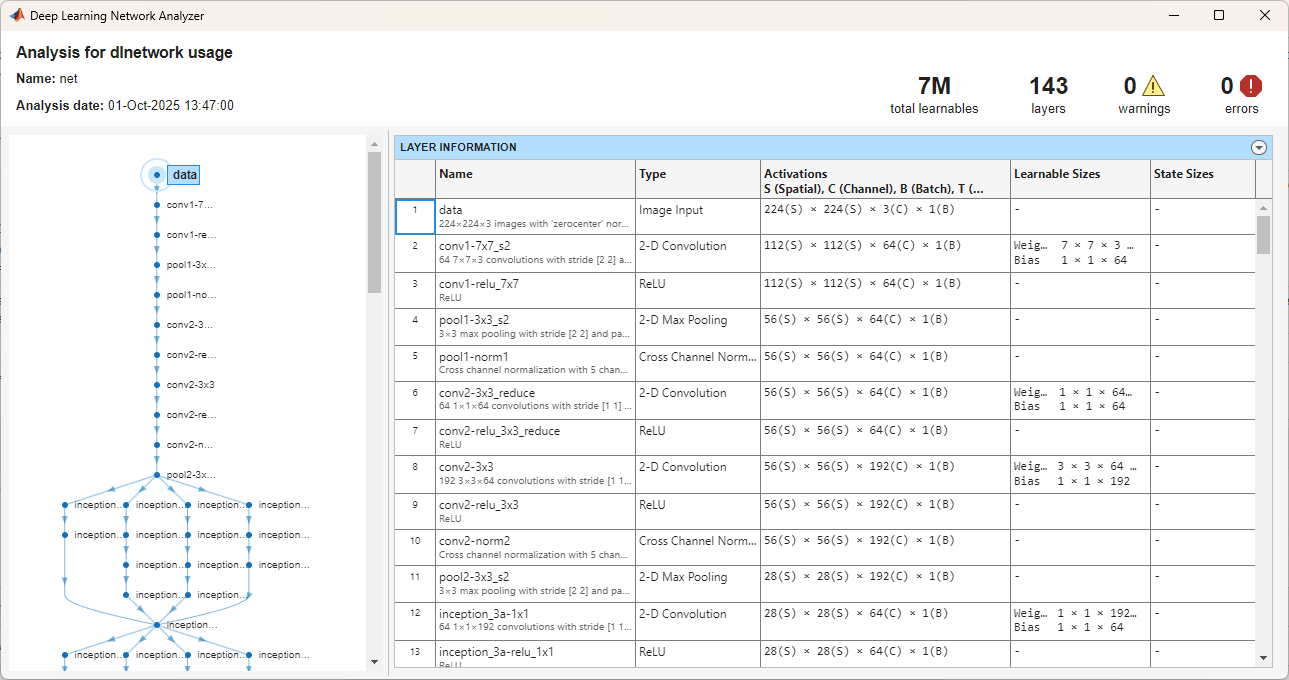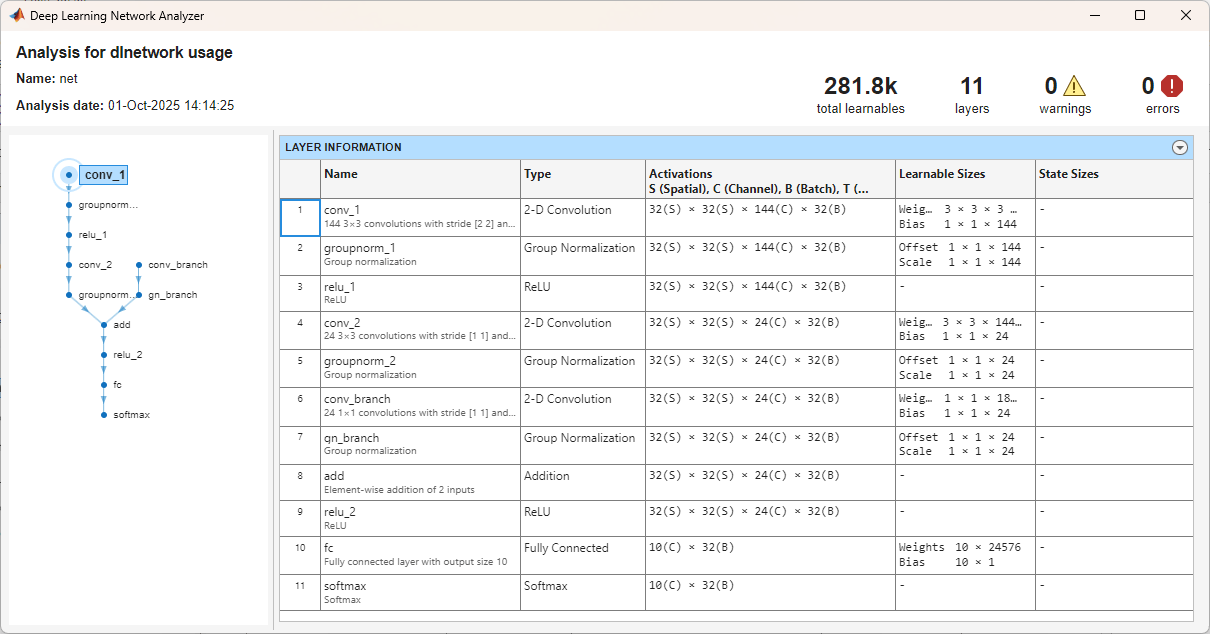analyzeNetwork

Analyze deep learning network architecture

Description

Use analyzeNetwork to visualize and understand the architecture of a network, check that you have defined the architecture correctly, and detect problems before training. Problems that analyzeNetwork detects include missing or unconnected layers, incorrectly sized layer inputs, an incorrect number of layer inputs, and invalid graph structures.

Tip

To interactively visualize, analyze, and train a network, use deepNetworkDesigner(net). For more information, see Deep Network Designer.

Trained Networks

example

analyzeNetwork(net) analyzes the SeriesNetwork or DAGNetwork object net. The function displays an interactive visualization of the network architecture and provides detailed information about the network layers. The layer information includes the number and sizes of layer activations, learnable parameters, and state parameters.

Network Layers

example

analyzeNetwork(layers) analyzes the network layers specified in layers and also detects errors and issues for trainNetwork workflows. layers can be a Layer array or a LayerGraph object. The function displays an interactive visualization of the network architecture and provides detailed information about the network layers. The layer information includes the number and sizes of layer activations, learnable parameters, and state parameters.

example

analyzeNetwork(layers,'TargetUsage',target) analyzes the network layers specified in layers for the specified target workflow. Use this syntax when analyzing a Layer array or layer graph for dlnetwork workflows.

analyzeNetwork(layers,dlX1,...,dlXn,'TargetUsage','dlnetwork') analyzes the network layers using example networks inputs dlX1,...,dlXn. The software propagates the example inputs through the network to determine the number and sizes of layer activations, learnable parameters, and state parameters. Use this syntax to analyze a network that has one or more inputs that are not connected to an input layer.

dlnetwork Objects

analyzeNetwork(dlnet) analyzes the dlnetwork object for custom training loop workflows. The function displays an interactive visualization of the network architecture and provides detailed information about the network layers. The layer information includes the number and sizes of layer activations, learnable parameters, and state parameters.

analyzeNetwork(dlnet,dlX1,...,dlXn) analyzes the dlnetwork object using example networks inputs dlX1,...,dlXn. The software propagates the example inputs through the network to determine the number and sizes of layer activations, learnable parameters, and state parameters. Use this syntax to analyze an uninitialized dlnetwork that has one or more inputs that are not connected to an input layer.

Examples

collapse all

net =
DAGNetwork with properties:

Layers: [144×1 nnet.cnn.layer.Layer]
Connections: [170×2 table]
InputNames: {'data'}
OutputNames: {'output'}

Analyze the network. analyzeNetwork displays an interactive plot of the network architecture and a table containing information about the network layers.

Investigate the network architecture using the plot to the left. Select a layer in the plot. The selected layer is highlighted in the plot and in the layer table.

In the table, view layer information such as layer properties, layer type, and sizes of the layer activations and learnable parameters. The activations of a layer are the outputs of that layer.

Select a deeper layer in the network. Notice that activations in deeper layers are smaller in the spatial dimensions (the first two dimensions) and larger in the channel dimension (the last dimension). Using this structure enables convolutional neural networks to gradually increase the number of extracted image features while decreasing the spatial resolution.

Show the total number of learnable parameters in each layer by clicking the arrow in the top-right corner of the layer table and select Total Learnables. To sort the layer table by column value, hover the mouse over the column heading and click the arrow that appears. For example, you can determine which layer contains the most parameters by sorting the layers by the total number of learnable parameters.

analyzeNetwork(net)Create a simple convolutional network with shortcut connections. Create the main branch of the network as an array of layers and create a layer graph from the layer array. layerGraph connects all the layers in layers sequentially.

layers = [
imageInputLayer([32 32 3])

reluLayer('Name','relu_1')

reluLayer

reluLayer

fullyConnectedLayer(10)
softmaxLayer
classificationLayer];

lgraph = layerGraph(layers);

Create the shortcut connections. One of the shortcut connections contains a single 1-by-1 convolutional layer skipConv.

skipConv = convolution2dLayer(1,16,'Stride',2,'Name','skipConv');

Analyze the network architecture. analyzeNetwork finds four errors in the network.

analyzeNetwork(lgraph)Investigate and fix the errors in the network. In this example, the following issues cause the errors:

• The skipConv layer is not connected to the rest of the network. It should be a part of the shortcut connection between the add1 and add2 layers. To fix this error, connect add1 to skipConv and skipConv to add2.

• The add2 layer is specified to have three inputs, but the layers only has two inputs. To fix the error, specify the number of inputs as 2.

• All the inputs to an addition layer must have the same size, but the add1 layer has two inputs with different sizes. Because the conv_2 layer has a 'Stride' value of 2, this layer downsamples the activations by a factor of two in the first two dimensions (the spatial dimensions). To resize the input from the relu2 layer so that it has the same size as the input from relu1, remove the downsampling by setting the 'Stride' value of the conv_2 layer to 1.

Apply these modifications to the layer graph construction from the beginning of this example and create a new layer graph.

layers = [
imageInputLayer([32 32 3])

reluLayer('Name','relu_1')

reluLayer

reluLayer

fullyConnectedLayer(10)
softmaxLayer
classificationLayer];

lgraph = layerGraph(layers);

skipConv = convolution2dLayer(1,16,'Stride',2,'Name','skipConv');

Analyze the new architecture. The new network does not contain any errors and is ready to be trained.

analyzeNetwork(lgraph)Create a layer graph for a custom training loop. For custom training loop workflows, the layer graph must not have an output layer.

layers = [
imageInputLayer([28 28 1],'Normalization','none','Name','input')
convolution2dLayer(5, 20,'Name','conv1')
batchNormalizationLayer('Name','bn1')
reluLayer('Name','relu1')
batchNormalizationLayer('Name','bn2')
reluLayer('Name','relu2')
batchNormalizationLayer('Name','bn3')
reluLayer('Name','relu3')
fullyConnectedLayer(10,'Name','fc')
softmaxLayer('Name','softmax')];

lgraph = layerGraph(layers);

Analyze the layer graph using the analyzeNetwork function and set the 'TargetUsage' option to 'dlnetwork'.

analyzeNetwork(lgraph,'TargetUsage','dlnetwork')Here, the function does not report any issues with the layer graph.

To analyze a network that has an input that is not connected to an input layer, you can provide example network inputs to the analyzeNetwork function. You can provide example inputs when you analyze dlnetwork objects, or when you analyze Layer arrays or LayerGraph objects for custom training workflows using the 'TargetUsage','dlnetwork' name-value option.

Define the network architecture. Construct a network with two branches. The network takes two inputs, with one input per branch. Connect the branches using an addition layer.

numFilters = 24;
inputSize = [64 64 3];

layersBranch1 = [
imageInputLayer(inputSize,'Normalization','none','Name','input')
groupNormalizationLayer('all-channels','Name','gn1Branch1')
reluLayer('Name','relu1Branch1')
groupNormalizationLayer('channel-wise','Name','gn2Branch1')
reluLayer('Name','reluCombined')
fullyConnectedLayer(10,'Name','fc')
softmaxLayer('Name','sm')];

layersBranch2 = [
convolution2dLayer(1,numFilters,'Name','convBranch2')
groupNormalizationLayer('all-channels','Name','gnBranch2')];

lgraph = layerGraph(layersBranch1);

Create the dlnetwork. Because this network contains an unconnected input, create an uninitialized dlnetwork object by setting the 'Initialize' name-value option to false.

dlnet = dlnetwork(lgraph,'Initialize',false);

Create example network inputs of the same size and format as typical inputs for this network. For both inputs, use a batch size of 32. Use an input of size 64-by-64 with three channels for the input to the layer 'input'. Use an input of size 64-by-64 with 18 channels for the input to the layer 'convBranch2'.

exampleInput = dlarray(rand([inputSize 32]),'SSCB');
exampleConvBranch2 = dlarray(rand([32 32 18 32]),'SSCB');

Examine the Layers property of the network to determine the order in which to supply the example inputs.

dlnet.Layers
ans =
12×1 Layer array with layers:

1   'input'          Image Input           64×64×3 images
2   'conv1Branch1'   Convolution           144 3×3 convolutions with stride [2  2] and padding 'same'
3   'gn1Branch1'     Group Normalization   Group normalization
4   'relu1Branch1'   ReLU                  ReLU
5   'conv2Branch1'   Convolution           24 3×3 convolutions with stride [1  1] and padding 'same'
6   'gn2Branch1'     Group Normalization   Group normalization
8   'reluCombined'   ReLU                  ReLU
9   'fc'             Fully Connected       10 fully connected layer
10   'sm'             Softmax               softmax
11   'convBranch2'    Convolution           24 1×1 convolutions with stride [1  1] and padding [0  0  0  0]
12   'gnBranch2'      Group Normalization   Group normalization

Analyze the network. Provide the example inputs in the same order as the layers that require inputs appear in the Layers property of the dlnetwork. You must provide an example input for all network inputs, including inputs that are connected to an input layer.

analyzeNetwork(dlnet,exampleInput,exampleConvBranch2)Input Arguments

collapse all

Trained network, specified as a SeriesNetwork or a DAGNetwork object. You can get a trained network by importing a pretrained network (for example, by using the googlenet function) or by training your own network using trainNetwork.

Network layers, specified as a Layer array or a LayerGraph object.

For a list of built-in layers, see List of Deep Learning Layers.

Network for custom training loops, specified as a dlnetwork object.

Target workflow, specified as one of the following:

• 'trainNetwork' — Analyze layer graph for usage with the trainNetwork function. For example, the function checks that the layer graph has an output layer and no disconnected layer outputs.

• 'dlnetwork' — Analyze layer graph for usage with dlnetwork objects. For example, the function checks that the layer graph does not have any output layers.

Example network inputs, specified as formatted dlarray objects. The software propagates the example inputs through the network to determine the number and sizes of layer activations, learnable parameters, and state parameters.

Use example inputs when you want to analyze a network that has inputs that are unconnected to an input layer.

The order in which you must specify the example inputs depends on the type of network you are analyzing:

• Layer array — Provide example inputs in the same order that the layers that require inputs appear in the Layer array.

• LayerGraph — Provide example inputs in the same order as the layers that require inputs appear in the Layers property of the LayerGraph.

• dlnetwork — Provide example inputs in the same order as the inputs are listed in the InputNames property of the dlnetwork.

If a layer has multiple unconnected inputs, then example inputs for that layer must be specified separately in the same order as they appear in the layer’s InputNames property.

You must specify one example input for each input to the network, even if that input is connected to an input layer.

Introduced in R2018a